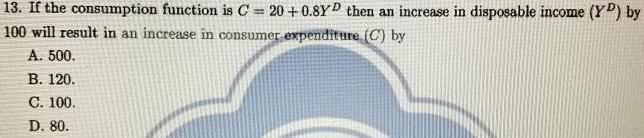Question:

# 13. If the consumption function is C=20+0.8Y^D then an

Last updated: 7/12/202213. If the consumption function is C=20+0.8Y^D then an increase in disposable income (Y^D) by 100 will result in an increase in consumer expenditure (C) by A. 500. B. 120. C. 100. D. 80.